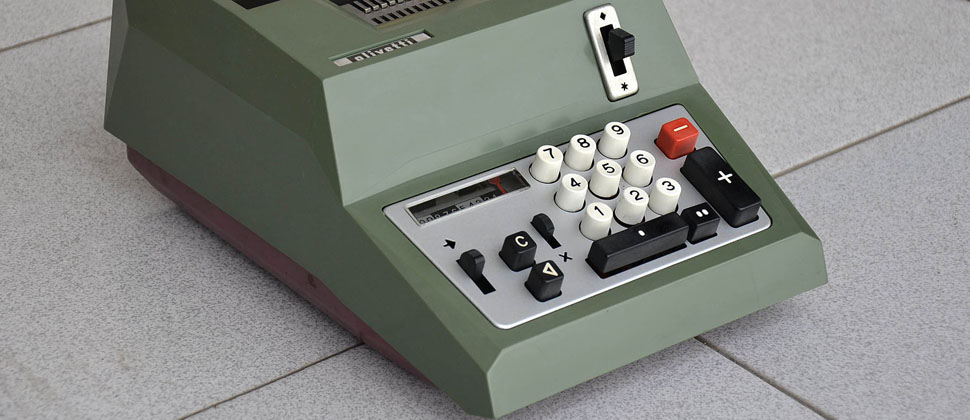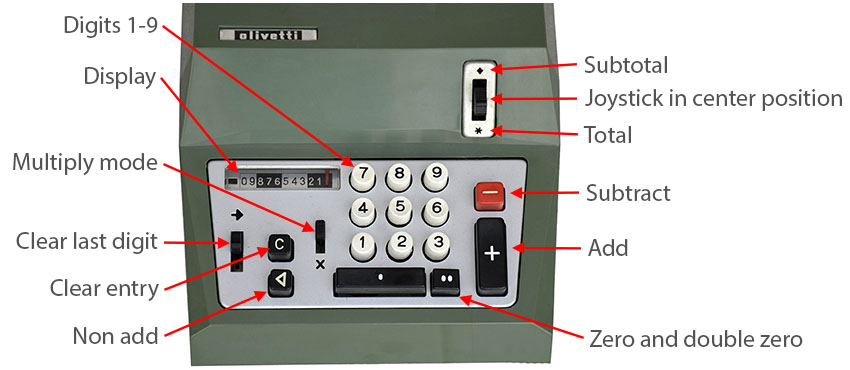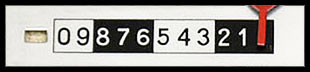Collection ItemOlivetti Summa Quanta 20EBrand: Olivetti.
Model: Summa Quanta 20E.
Origin: Ivrea, Italy. This example was built in Argentina. Olivetti was stablished in Argentina in 1932 and made a complete line of mechanical, electro-mechanical and electronic typewriters and calculators.
Introduction: 1970?.
Type: Basic printing electro-mechanical calculator.
Functions: Add and subtract. Multiply by repetitive adding. Subtotal and total. Non-add mode for printing additional data like item number, item order, etc..
Logic comments: Like the most of the basic calculators of that era, this calculator uses 2 mechanical registers:

Input Register (IR): Stores the keyboard input. Any digit you enter is appended on the right of the IR, the remaining digits are shifted one position to the left, or 2 positions if you press the double zero key. The number of actual digits in IR is shown on the display. This register have a precision of 10 digits in integer format with no decimals. The IR is automatically cleared after a normal add or subtract operation, except if you move the Multiply mode sliding knob to the "X" position. Also you can clear it by pressing the Clear entry key.

Accumulator Register (AR): Stores the result of a chain of adding and subtracting operations. The AR register have a precision of 11 digits with no decimals. This register is cleared when you move the joystick to the * (Total) position.

Principle of operation:
Whenever you press the + or - keys the IR register is added or subtracted, respectively, to the AR register, and the IR register is cleared, except if the Multiply Mode is on (see below).
When you move the joystick to the Subtotal position (up) the AR will be printed and the contents will remain, allowing to continue with more operations.
If you move the joystick to the Total position (down) the AR will be printed and the content will be cleared.
For example, to compute 12+23-34+45 you must enter:
12 +
23 +
34 -
45 +
Move the joystick to * (Total) position and the total 46 will be printed in red on the ticket paper roll.

Multiplication:
Although this calculator can not multiply in one operator step, you can do a multiplication by succesive adding.
I will explain a "basic", an "abbreviate" and an "expert" mode to do it.
For example, to compute 725 x 5 you can use this "basic" method:
- Clear the machine: Set the joystick to the Total position to clear all registers.
- Enter 725.
- Set the Multiply Mode sliding knob in "X" position. This avoids the IR to be cleared after pressing + or -.
- Now press the + key five times, the IR register will be added 5 times to the AR register.
- Print the result: Set again the joystick to the Total position and the result 3625 will be printed.

This method works fine if you multiply by a small number, 5 in this example, but what if you want to multiply 725 x 213? You can complete this operation by adding only 6 times using this "abbreviate" method:
- Clear the machine: Set the joystick to the Total position to clear all registers.
- Enter 725.
- Set the Multiply Mode sliding knob to the "X" position.
- Press the + key three times for the rightmost digit of 213.
- Press the Zero (0) key to shift the IR one position to the left and convert IR into 7250.
- Now press the + key one time for the digit "1" of 213.
- Again press the Zero (0) key to shift the IR one position to the left and convert IR into 72500.
- Press the + key two times for the digit "2" of 213.
- Set again the joystick to the Total position and the result 154425 will be printed.

725 +
725 +
725 +
7250 +
72500 +
72500 +

Please note that you pressed the + key only six (2+1+3) times.

Also you can use an "expert" mode to reduce further more the + key press in some cases.
For example, if you multiply 12866 x 897, you can compute 897 as 1000-100-3, so the sequence is:
- Clear the machine: Set the joystick to the Total position to clear all registers.
- Enter 12866.
- Set the Multiply Mode sliding knob to the "X" position.
- Press the - key three times to accumulate IR * -3.
- Press the Zero (0) key two times (or double-zero key) to shift the IR two positions to the left and convert it into 1286600.
- Press the - key one time to accumulate IR * -100.
- Press the Zero (0) key to shift the IR one position to the left and convert IR into 12866000.
- Now Press the + key one time to accumulate IR * 1000.
- Set again the joystick to the Total position and the result 11540802 will be printed.

12866 -
12866 -
12866 -
1286600 -
12866000 +

Please compare the 3 methods described for the multiplication:
If you multiply 12866 x 897 you need to press the + and - keys...
- Using the "basic" mode: 897 times.
- Using the "abbreviate" mode: 24 times (8+9+7).
- Using the "expert" mode: Only 5 times! (1+1+3).
Keyboard: Keyboard layout:Digits 1-9: This calc accepts a maximum of 10 digits including the zeroes.
Zero and double zero: one and two zeroes, the calc does not accept input leading zeroes.
Subtract: Subtracts the IR to the AR.
Multiply mode: Set into the "X" position to avoid clearing the IR and allow to multiply by repetitive addition.
Clear entry: Clears the input register (IR).
Clear last digit: Clears the last digit entered into IR, the red needle in the display will move one position to the right. Non add: The input register IR is printed but not added or subtracted from the accumulator register (AR).
Display: See description bellow.
Subtotal: The AR is printed and the content is not cleared to allow futher operations.
Center (normal) position: Normal position of the joystick.
Total: The AR is printed and the content cleared to begin a new series of operations.
Display: This simple display shows the number of digits you entered from the keyboard into the IR.
After a digit 0-9 is pressed the red needle will be desplaced one position to the left, or two positions if you press the double zero key. After pressing +, -, C keys or Subtotal or Total joystick position the red needle will move to the right.
The small rectangle on the left indicates when the result in AR is negative.In this case there are no digits into the IR.
Printer: The printer have a precisión of 11 digits plus an indicator of Total, Subtotal and Negative result.
The printer uses a 2-color ink ribbon with black color for normal input and red color for total and subtotal.
Printing example:The red line with diamond indicates Subtotal and the red line with asterisk is the Total.
Size: 11.02 x 7.28 x 4.72 in, 280 x 185 x 120 mm.
Accesories: Dechatable power cord and soft case.
Condition: (9/10) Very good near mint, almost no marks on the casing, tested and fully working..

 Some advertisements of the Summa Quanta 20E I found on the web, click on the thumbnail.

 Pictures of my Summa Quanta 20E.

 Pictures taken with